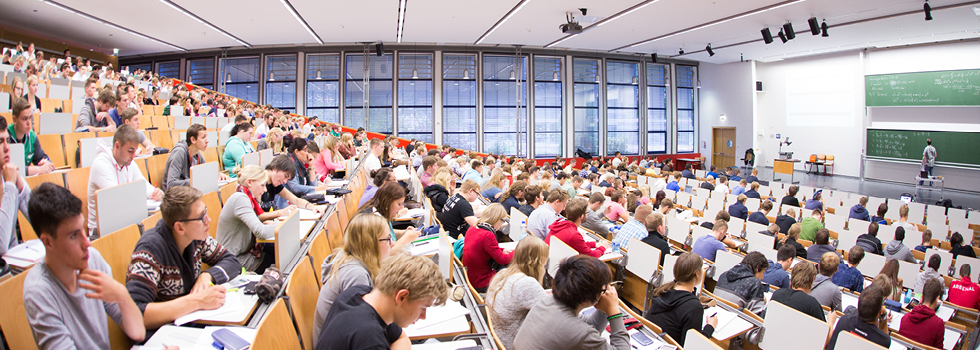••## Mathematics

#### Brief Profile

As one of the oldest sciences, in the 21st century Mathematics still provides an essential foundation for almost every area of science, engineering, economics and management. In terms of research and teaching the Faculty sees its responsibility as lying both in the training of highly-qualified graduates to find solutions to the technological and social challenges of a modern society as well as in the use-based application of research. Highly topical problems in mathematics demonstrate its essential nature. For example, groups of researchers are working on developing algorithms for the personalised medicine of the future or the Travelling Salesman Problem, where the shortest route through certain cities has to be found using modern mathematical methods.

The Faculty sees mathematics as a science that transcends individual disciplines and accordingly strongly supports research in the key research priority area of "Dynamic Systems: Biosystems Engineering". Furthermore it's involved in the International Max Planck Research School (IMPRS). Primarily there are joint research projects and publications on mathematical modelling, optimisation and numerics with the engineering and biomedical sciences departments. The Faculty also cooperates closely with the mathematical working group at the Max Planck Institute.

#### Research topics

• Discrete Mathematics and Optimisation – e.g. algebra, algebraic statistics, coding theory/cryptography, discrete/convex geometry, finite fields
• Non-Linear Analysis and Numerics – e.g. qualitative solution characteristics of elliptical, parabolic and hyperbolic differential equations; differential geometric questions; stability and accuracy analyses of discretisations
• Stochastics – e.g. probability theory and mathematical stochastics

#### Institute

The Faculty of Mathematics is home to the following institutes:

Last Modification: 23.06.2021 - Contact Person: